# Nanoindentation for Measurement of Stress-Strain

The Stress-Strain curve achieved by Nanoindentation shows the correlation of "Stress" and "Strain" of a specified material under nano controlled loads. The Nanoindentation method provides important Stress-Strain curve data at a nano level scale without the large and intense equipment, unlike the conventional Tensile Testing method of achieving Stress-Strain curve data, which provides data at a macro level. The Stress-Strain curve of different materials will vary widely, and this eventually provides vital information on the threshold between the plastic-elastic and elastic behavior area as the sample is subject to increasing loads.

## Importance of Nanoindentation for QC and R&D Applications

Stress-Strain data is important to study the bulk material’s surface characteristic. Nanoindentation offers a user-friendly and reliable method to quickly study stress-strain data. In addition, the measurement of stress-strain behavior on the nano scale opens the door for studying vital properties on particles and coatings in composite materials among others. Therefore, Nanoindentation can give information on yield strength and elastic limit, in addition to fracture toughness, creep information, elastic modulus, hardness, etc.

## Measurement Objective

In this application, the Nanovea Mechanical Tester is employed to study Elastic Modulus and Hardness vs. depth and Stress-Strain data on a normal steel sample, which was selected for its widely recognized Stress-Strain curve to show the accuracy and control of the nano scale Stress-Strain data. For the test, a spherical tip of 5 μm in radius was used because it could reach higher stresses than the elastic limit for steel.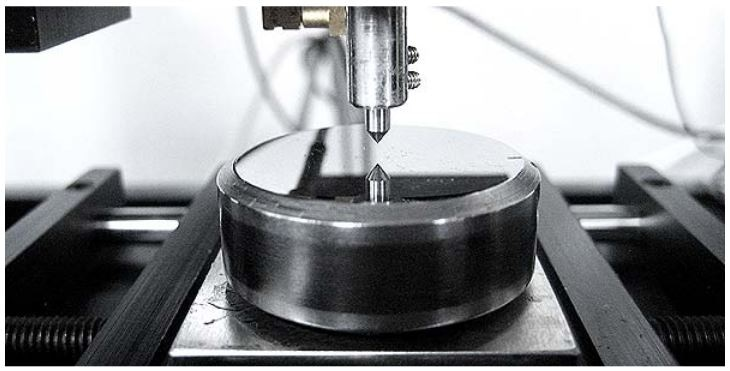## Measurement Principle

Nanoindentation is based on the ISO 14577 and ASTM E2546 standards for instrumented indentation. It employs a well-known method where an indenter tip with a recognized geometry is driven into a particular site of the material to be tested, by using an increasing normal load. While reaching a pre-set maximum value, the normal load is decreased until complete relaxation occurs. A piezo actuator is used to apply the load, which is measured in a controlled loop by means of a high sensitivity load cell. The position of the indenter in relation to the sample surface is precisely monitored through high precision capacitive sensor during the experiment.

The data specific to the mechanical nature of the material under test are provided by the resulting load/displacement curves. For such data, established models are employed to calculate modulus values and quantitative hardness. Nanoindentation is particularly suitable for measuring the load and penetration depth at nanometer scales and has the following specifications:

• Maximum displacement (Dual Range): 50 µm or 250 µm
• Depth Resolution (Theoretical): 0.003 nm
• Depth Resolution (Noise Level): 0.05 nm
• Maximum force: 400 mN
• Load Resolution (Theoretical): 0.03 µN
• Load Resolution (Noise Floor): 1.5 µN

### Analysis of Indentation Curve

Following the ASTM E2546 (ISO 14577), elastic modulus and hardness are determined via load/displacement curve as for the example below.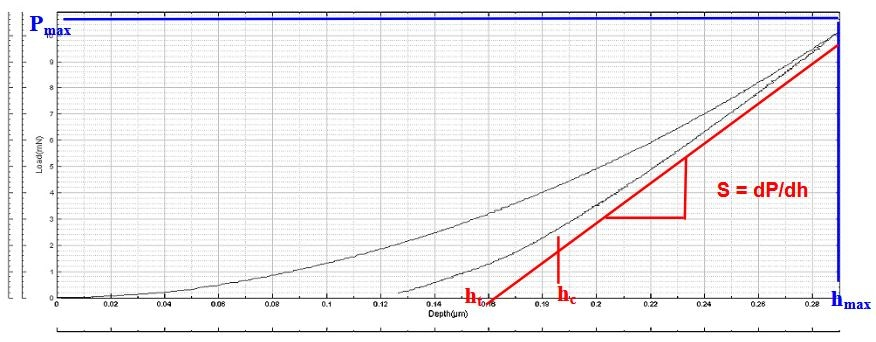Hardness

The hardness is determined from the maximum load, Pmax, divided by the projected contact area, Ac: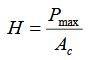Young’s Modulus

The reduced modulus, Er, is given by: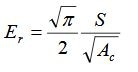This can be determined having derived S and Ac from the indentation curve using the area function, Ac being the projected contact area. Then, the Young’s modulus, E, can be achieved from: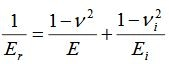Where Ei and νi are the Young’s modulus and Poisson coefficient of the indenter and ν is the Poisson coefficient of the tested sample.

How are these calculated?

A power-law fit via the upper 1/3 to 1/2 of the unloading data intersects the depth axis at ht. The stiffness, S, is given by the slope of this line. Then, the contact depth, hc, is calculated as: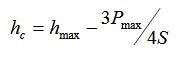The indenter area function is evaluated to calculate the contact Area Ac. This function will rely on the diamond geometry and at low loads by an area correction.

The area function is Ac =24.5hc2 for a perfect Berkovich and Vickers indenters; the area function is Ac=2.60hc2 for Cube Corner indenter; and the area function is Ac=2πRhc for Spherical indenter where R is the radius of the indenter. As mentioned before, the elastic components can be modeled as springs of elastic constant E, given the formula: σ= Eε

where σ is the stress, E is the elastic modulus of the material, and ε is the strain that takes place under the given stress, akin to Hooke's Law. It is possible to model the viscous components as dashpots such that the stress-strain rate relationship can be given as,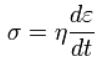where σ is the stress, η is the viscosity of the material, and dε/dt is the time derivative of strain.

The analysis highly depends on the model that is chosen. Nanovea offers the tool to collect the data of displacement against depth during the creep time. The software gives the maximum creep displacement against the maximum depth of indent and the average speed of creep in nm/s. Creep can be best studied during quicker loading. It would be better to use a spherical tip.

Other tests possibly include the following:

Volume of indent, Work of Indentation, Visco Elastic, Plastic and Elastic Work, Compression, Creep and others.

## Test Conditions and Procedures

The following indentation parameters were used:

. .
Maximum force (mN) 300
Creep (s) 0
Computation Method ASTM E-2546 & Martens Hardness
Indenter type 5 micron Spherical tip
# Cycles 8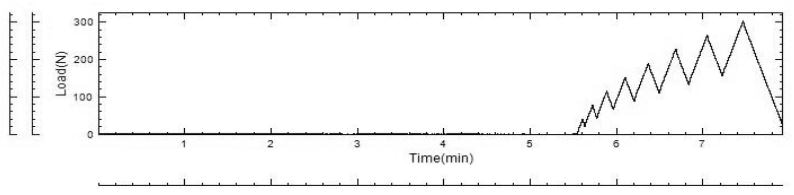Loading Versus Time Curve that was applied for this test

## Results

The increase in load cycling at the same position offers the following depth versus load curve where each cycle is well identified.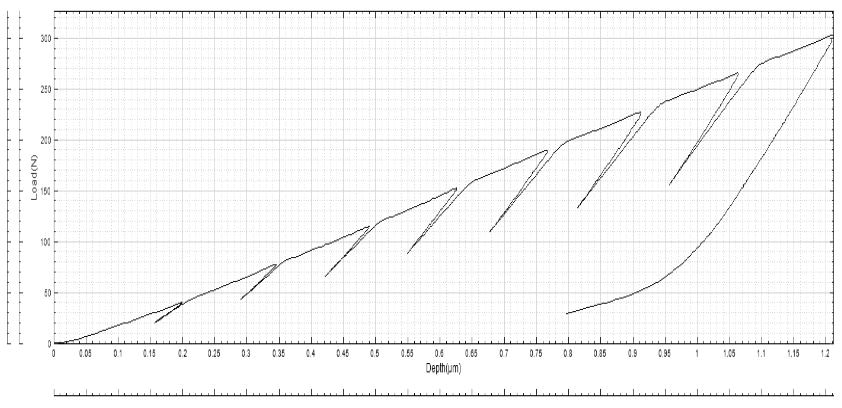Each cycle can be regarded as being one indent done at its own depth. Hence, hardness and elastic modulus vs. depth can be directly plotted from the data acquired for each cycle.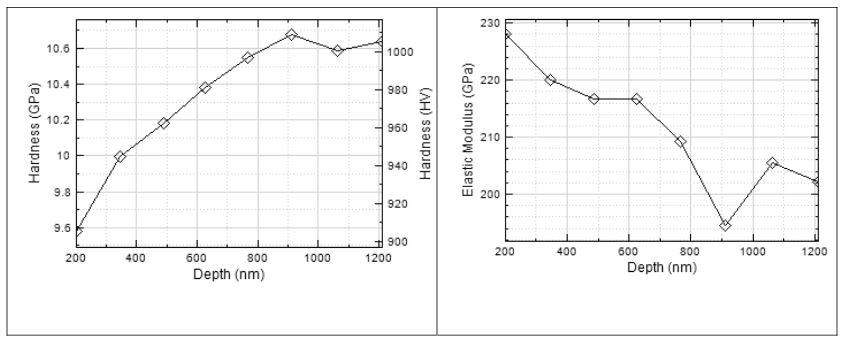Stress versus strain can be plotted from the data acquired at each cycle. The maximum depth at each cycle and the maximum load applied at each cycle help to calculate the true maximum stress applied to each particular cycle. Strain is measured from the residual depth at each cycle which, in turn, is determined from the partial unloading. This helps to calculate the radius of the remaining imprint which is divided by the radius of the tip used to provide the strain factor.

Cycle # Load [ mN ] Max Depth [ nm ] Stress [ GPa ] Residual Depth [ nm ] Strain
1 40.34 193 2661 70 0.166745
2 77.4 339 2907 206 0.283947
3 115.03 482 3038 278 0.328799
4 152.44 619 3135 414 0.398427
5 189.8 759 3183 507 0.438769
6 227.18 906 3192 646 0.491637
7 265.61 1055 3205 727 0.519287
8 302.65 1203 3203 858 0.560137

An important set of data related to the material under test is obtained by plotting the stress vs. strain. First, the elastic and plastic zone can be clearly seen and the stress at this transition point can be measured. It should be noted that 0.4 for strain is a value that is typically found to be consistent with a fully developed plastic zone. For this sample, 3150 GPa can be considered as the elastic limit.## Conclusion

To sum up, this article has shown how the Nanovea Mechanical Tester, in Nanoindentation Mode, can be employed to provide consistent stable stress-strain data. The use of a spherical tip as well as increased cyclic loads makes it possible to measure the properties of the material under increased stress. Nanoindentation provides stress-strain data which help to identify the material’s elastic limit. It musts be remembered that the data was collected from the first 1.2 micron of the surface. The radius of probe and the loads can be changed to test different materials at controlled depths. The Nanovea Mechanical Tester is capable of providing these tests from the sub mN range to 200N.

It has also been shown that the increasing load cycles can be used to determine the mechanical properties vs. depth. Hardness and elastic modulus versus depth are the main parameters. This can be extremely useful in determining case hardening and would prevent the need for preparing a particular cut sample by simply requiring a single indent rather than multiple ones. At low load, it may also be carried out on the end product because the indent is so small that it cannot be viewed by customers. The disadvantage is that it would be difficult to compare the hardness data with the traditional case hardening data. The change of hardness vs. depth trend will however match with the traditional case hardening data.

In the case of thin and thick coatings, the multi-cycle test can be quite useful in measuring hardness and elastic modulus vs. depth. For these tests, a sharper Berkovich or Vickers tip would be suggested. It should also be remembered that the multi-cycle test can be carried out at constant load. This can be useful for studying fatigue in certain materials or for eliminating the effect of porosity in specific materials such as bones to acquire the true elastic modulus.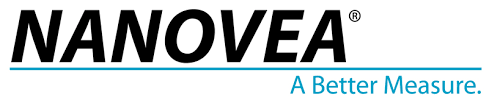This information has been sourced, reviewed and adapted from materials provided by Nanovea.

## Citations

Please use one of the following formats to cite this article in your essay, paper or report:

• APA

Nanovea. (2019, May 22). Nanoindentation for Measurement of Stress-Strain. AZoNano. Retrieved on September 20, 2019 from https://www.azonano.com/article.aspx?ArticleID=4709.

• MLA

Nanovea. "Nanoindentation for Measurement of Stress-Strain". AZoNano. 20 September 2019. <https://www.azonano.com/article.aspx?ArticleID=4709>.

• Chicago

Nanovea. "Nanoindentation for Measurement of Stress-Strain". AZoNano. https://www.azonano.com/article.aspx?ArticleID=4709. (accessed September 20, 2019).

• Harvard

Nanovea. 2019. Nanoindentation for Measurement of Stress-Strain. AZoNano, viewed 20 September 2019, https://www.azonano.com/article.aspx?ArticleID=4709.

## Ask A Question

Do you have a question you'd like to ask regarding this article?